# charles' LAW.pptx

teacher at deped
May. 30, 2023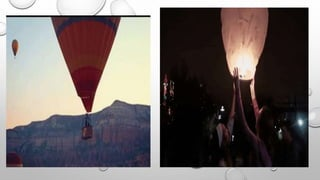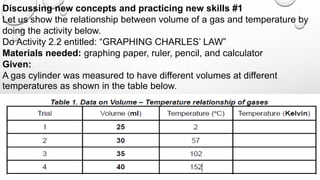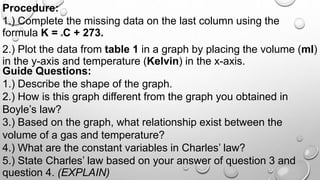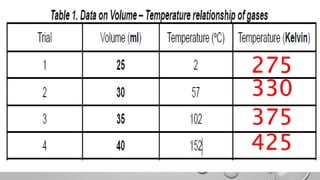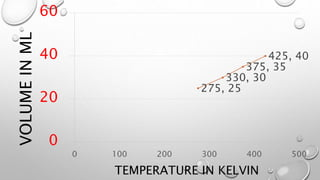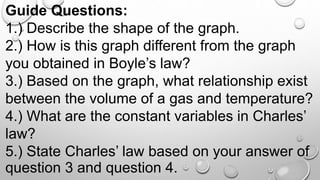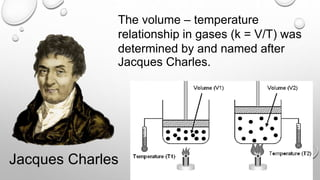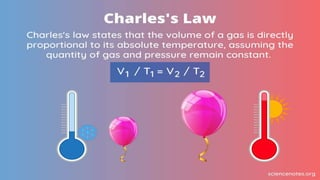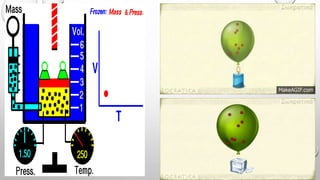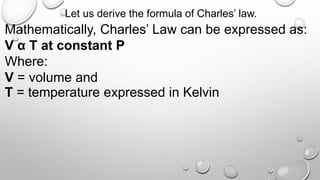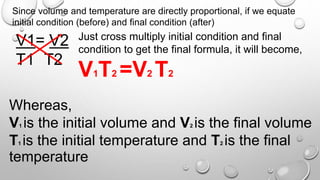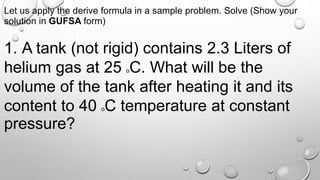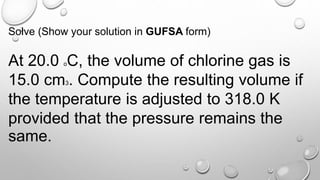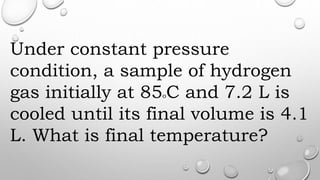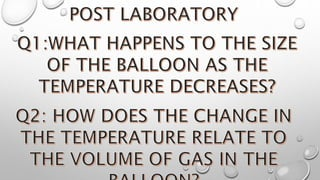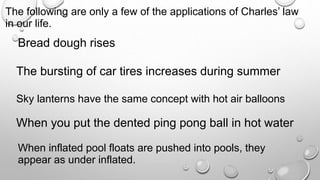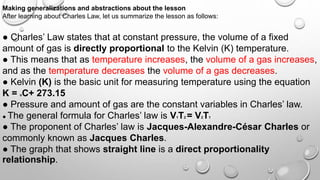1 of 17

### charles' LAW.pptx

• 2. Discussing new concepts and practicing new skills #1 Let us show the relationship between volume of a gas and temperature by doing the activity below. Do Activity 2.2 entitled: “GRAPHING CHARLES’ LAW” Materials needed: graphing paper, ruler, pencil, and calculator Given: A gas cylinder was measured to have different volumes at different temperatures as shown in the table below.
• 3. 2.) Plot the data from table 1 in a graph by placing the volume (ml) in the y-axis and temperature (Kelvin) in the x-axis. Guide Questions: 1.) Describe the shape of the graph. 2.) How is this graph different from the graph you obtained in Boyle’s law? 3.) Based on the graph, what relationship exist between the volume of a gas and temperature? 4.) What are the constant variables in Charles’ law? 5.) State Charles’ law based on your answer of question 3 and question 4. (EXPLAIN) Procedure: 1.) Complete the missing data on the last column using the formula K = oC + 273.
• 5. 275, 25 330, 30 375, 35 425, 40 0 20 40 60 0 100 200 300 400 500 VOLUME IN ML TEMPERATURE IN KELVIN
• 6. Guide Questions: 1.) Describe the shape of the graph. 2.) How is this graph different from the graph you obtained in Boyle’s law? 3.) Based on the graph, what relationship exist between the volume of a gas and temperature? 4.) What are the constant variables in Charles’ law? 5.) State Charles’ law based on your answer of question 3 and question 4.
• 7. Jacques Charles The volume – temperature relationship in gases (k = V/T) was determined by and named after Jacques Charles.
• 10. Let us derive the formula of Charles’ law. Mathematically, Charles’ Law can be expressed as: V α T at constant P Where: V = volume and T = temperature expressed in Kelvin
• 11. Since volume and temperature are directly proportional, if we equate initial condition (before) and final condition (after) V1= V2 T1 T2 Just cross multiply initial condition and final condition to get the final formula, it will become, V1T2 =V2 T2 Whereas, V1 is the initial volume and V2 is the final volume T1 is the initial temperature and T2 is the final temperature
• 12. Let us apply the derive formula in a sample problem. Solve (Show your solution in GUFSA form) 1. A tank (not rigid) contains 2.3 Liters of helium gas at 25 oC. What will be the volume of the tank after heating it and its content to 40 oC temperature at constant pressure?
• 13. Solve (Show your solution in GUFSA form) At 20.0 oC, the volume of chlorine gas is 15.0 cm3. Compute the resulting volume if the temperature is adjusted to 318.0 K provided that the pressure remains the same.
• 14. Under constant pressure condition, a sample of hydrogen gas initially at 85oC and 7.2 L is cooled until its final volume is 4.1 L. What is final temperature?
• 16. The following are only a few of the applications of Charles’ law in our life. Bread dough rises The bursting of car tires increases during summer Sky lanterns have the same concept with hot air balloons When you put the dented ping pong ball in hot water When inflated pool floats are pushed into pools, they appear as under inflated.
• 17. Making generalizations and abstractions about the lesson After learning about Charles Law, let us summarize the lesson as follows: ● Charles’ Law states that at constant pressure, the volume of a fixed amount of gas is directly proportional to the Kelvin (K) temperature. ● This means that as temperature increases, the volume of a gas increases, and as the temperature decreases the volume of a gas decreases. ● Kelvin (K) is the basic unit for measuring temperature using the equation K = oC+ 273.15 ● Pressure and amount of gas are the constant variables in Charles’ law. ● The general formula for Charles’ law is V1T2 = V2T1 ● The proponent of Charles’ law is Jacques-Alexandre-César Charles or commonly known as Jacques Charles. ● The graph that shows straight line is a direct proportionality relationship.

### Editor's Notes

1. Have you seen a hot air balloon or a sky lantern rises in the sky? What causes them to rise in the sky? What gas law operates the concept of hot air balloon and sky lantern?
2. In his experiment, Jacques Charles trapped a sample of gas in a cylinder with a movable piston in a water bath at different temperatures. Jacques Charles found out that different gases decreased their volume by factors 1/273 per oC of cooling. With this rate of reduction, if gas will be cooled up to -273 oC, it will have zero volume.
3. When the temperature is decreased, the volume is also decreased. This is because the kinetic energy of the gas particles is affected. Lowering the temperature Causes the gas particles to move slowly and results in fewer collisions and so it will occupy smaller space. Why is there a need to convert oC to Kelvin (K)? Kelvin is the basic unit for measuring temperature in the International System. “It denotes the absolute temperature scale whereby zero (0) K or absolute zero is defined as the temperature when molecules will have the lowest energy”.
4. Bread dough rises because yeast produces carbon dioxide. When placed in an oven, the heat causes the gas to expand and the bread rises even further. because the volume increases in a tire when it’s hot and small, and so the pressure increases in a hot tire is significant. In addition, high temperature causes rubber to weaken, which increases the chance that the tire will pop. As the temperature inside the sky lantern increases the volume of air also increases causing the sky lantern to float in the atmosphere. the temperature of the gas increases because the water on the outside is heating the gas (or air) inside. Charles’ law states that when a gas’ temperature is increased; it will take up more space so a dented ball can be fixed using hot water. The pool floats shrink because the temperature of the pool is lower than the surrounding atmosphere.